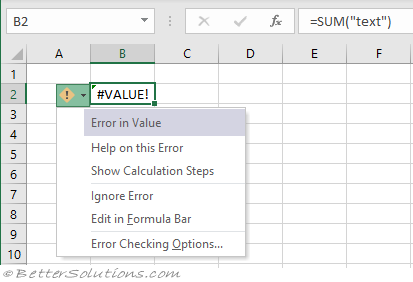# Result In An Error

This rule will identify cells that contain formulas that result in errors.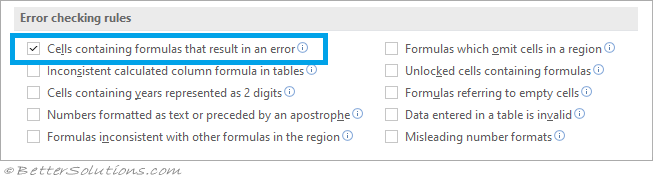Possible errors include:
#DIV/0! - Divide by Zero Error
#N/A - Value Not Available Error
#NAME? - Invalid Name Error
#NULL! - Null Error
#NUM! - Number Error
#REF! - Invalid Cell Reference Error
#VALUE! - Error in Value

### Example - Divide by Zero Error

When the a formula tries to divide by zero.
Enter the formula "=10/0" into cell "B2".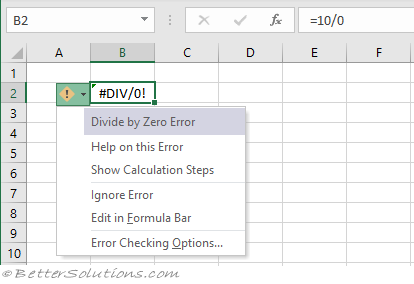Help on this Error - Displays the Microsoft Excel help window with possible causes and solutions for correcting this type of error.
Show Calculation Steps - Displays the Evaluate Formula dialog box.
Ignore Error - Permanently removes the smart tag from the cell. You can press F2 and Enter to bring the smart tag back.
Edit in Formula Bar - Takes you to the formula bar so you can edit the formula.
Error Checking Options - Displays the Options, Formulas Tab.

When a formula contains something that does not make sense.
Enter the formula "=A1.A2".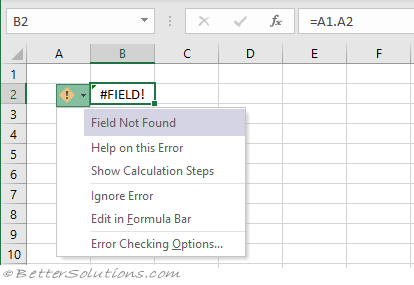### Example - Value Not Available Error

When a function contains an invalid argument.
Enter the formula "=CONVERT(1,,)".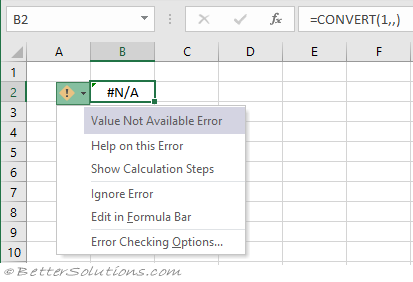### Example - Invalid Name Error

When a formula contains a named range that does not exist.
Enter the formula "=thisone" into cell "B2".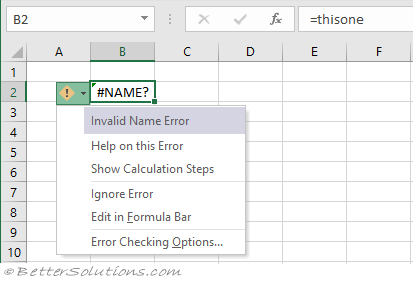### Example - Null Error

When a function contains an invalid range separator.
Enter the formula "=AREAS(C1 C2)" into cell "B2".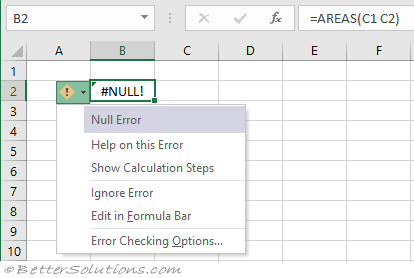### Example - Number Error

When a formula contains numeric values that are not valid.
Enter the formula "A1^A2" into cell "B2".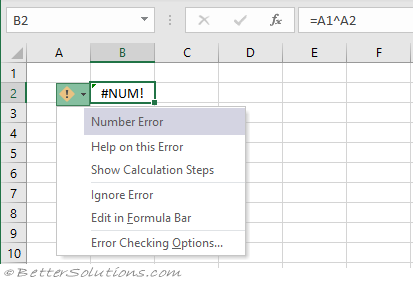### Example - Invalid Cell Reference Error

When a formula references a cell that has been deleted.
Enter the number 10 into cell "B2".
Enter the formula "=B2" into cell "B3".
Delete row 2.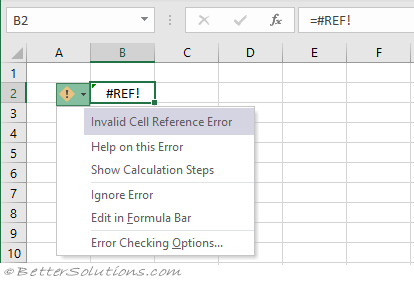### Example - Error in Value

When a function contains an invalid argument.
Enter the formula "=SUM("text")" into cell "B2".